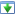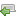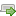Choose a version here. If you've not already started the tutorial, just go with the latest one.

 30 Aug 2014 Preview version Choose 5 Oct 2014 Explicitly adds a specific charset for htmlspecialchars(), and wraps it with a custom function Choose 16 Oct 2014 Updated redirect function to work with vhost subfolders Choose 4 Nov 2014 Minor improvements: added missing docblock, fixed security issue, CSS tweak. Switched hashing method to DEFAULT instead of BCRYPT, this is best practice. Choose ♥ 25 Nov 2014 Improve the notes on getting started, in particular choosing a programmer's editor. Added introduction to mod_rewrite rules. Choose 16 Aug 2018 Some bug fixes, remove compatibility library for earlier version of PHP Choose
OK
NB: There are several versions of this tutorial, each successive one containing additional improvements. If you're in the middle of working through it, please check the versions panel above, to ensure you're not mixing code from different versions.

## Using real data

Since we now have some data in our database, we can change our home page to render that data, rather than the mock data it has now. It's worth noting though that the process of designing the page with dummy data hasn't been a waste of time; using a mock-up is often a valuable part of deciding what a page should contain, and where those elements should go. So, make these changes next:` `
` `
` `
` `
` `
` `
` `
` `
` `
` `
` `
` `
` `
` `
` `
` `
` `
` `
` `
` `
` `
` `
` `
`1`
`2`
`3`
`31`
`32`
`33`
`34`
`35`
`36`
`37`
` `
` `
` `
` `
` `
` `
`38`
`39`
`40`
`41`
`42`
`43`
`44`
`<!DOCTYPE html>`
`<html>`
` <head>`
` <h1>Blog title</h1>`
` <p>This paragraph summarises what the blog is about.</p>`
` <?php for (\$postId = 1; \$postId <= 3; \$postId++): ?>`
` <h2>Article <?php echo \$postId ?> title</h2>`
` <div>dd Mon YYYY</div>`
` <p>A paragraph summarising article <?php echo \$postId ?>.</p>`
` <p>`
` <a href="#">Read more...</a>`
` </p>`
` <?php endfor ?>`
` </body>`
`</html>`
`1`
`2`
`3`
`4`
`5`
`6`
`7`
`8`
`9`
`10`
`11`
`12`
`13`
`14`
`15`
`16`
`17`
`18`
`19`
`20`
`21`
`22`
`23`
`24`
`25`
`26`
`31`
`32`
`33`
`34`
`35`
`36`
`37`
`38`
`39`
`40`
`41`
`42`
`43`
`44`
`45`
`46`
`47`
`48`
`49`
`50`
`<?php`
`// Work out the path to the database, so SQLite/PDO can connect`
`\$root = __DIR__;`
`\$database = \$root . '/data/data.sqlite';`
`\$dsn = 'sqlite:' . \$database;`
`// Connect to the database, run a query, handle errors`
`\$pdo = new PDO(\$dsn);`
`\$stmt = \$pdo->query(`
` 'SELECT`
` title, created_at, body`
` FROM`
` post`
` ORDER BY`
` created_at DESC'`
`);`
`if (\$stmt === false)`
`{`
` throw new Exception('There was a problem running this query');`
`}`
`?>`
`<!DOCTYPE html>`
`<html>`
` <head>`
` <h1>Blog title</h1>`
` <p>This paragraph summarises what the blog is about.</p>`
` <?php while (\$row = \$stmt->fetch(PDO::FETCH_ASSOC)): ?>`
` <h2>`
` <?php echo htmlspecialchars(\$row['title'], ENT_HTML5, 'UTF-8') ?>`
` </h2>`
` <div>`
` <?php echo \$row['created_at'] ?>`
` </div>`
` <p>`
` <?php echo htmlspecialchars(\$row['body'], ENT_HTML5, 'UTF-8') ?>`
` </p>`
` <p>`
` <a href="#">Read more...</a>`
` </p>`
` <?php endwhile ?>`
` </body>`
`</html>`

The first few lines determine the file path to the database, and then we create a new PDO object with `new PDO()`, which we can use to access the data. We then use the `query()` method to run a SQL statement that reads articles from the `post` table.

So, refresh your browser's view of `http://localhost/index.php`, and ensure that your new changes render the posts from the database without errors. I'll then explain what is happening.

After connecting to the database, the query is used to retrieve column values for each table row, returning ordering the rows in `created_at DESC` (i.e. in reverse creation order, or most recent first). It then enters a loop (in this case a `while()`) to render all the posts it finds.

We use `\$stmt->fetch()` to read the next available row, until the point when there are no rows left. When that happens, this method will return false and the loop will exit.

For every row, there are two observations worth making. Firstly, rows are returned in an array, so we access them using the array syntax such as `\$row['title']`. Secondly, you'll see that where text strings are output, they are wrapped in the `htmlspecialchars()` function. The reason for this is that, if user input (a blog title or blog post in this case) contains angle brackets, it could break the HTML used in the page layout, and worse, might let a user inject unauthorised JavaScript that would be run on other people's computers.

You'll notice that most of the PHP code to deal with the database side of things is in the top half of the file, and the second half is predominantly HTML. This isn't an accident: this arrangement creates a healthy separation between the two, which makes each easier to work with. Of course, there are a few cases where dynamic output is required inside the HTML, but these are kept deliberately short.

~

The next step is to create a page to show individual posts. Add the following file:`1`
`2`
`3`
`4`
`5`
`6`
`7`
`8`
`9`
`10`
`11`
`12`
`13`
`14`
`15`
`16`
`17`
`18`
`19`
`20`
`21`
`22`
`23`
`24`
`25`
`26`
`27`
`28`
`29`
`30`
`31`
`32`
`33`
`34`
`35`
`36`
`37`
`38`
`39`
`40`
`41`
`42`
`43`
`44`
`45`
`46`
`47`
`48`
`49`
`50`
`51`
`52`
`53`
`54`
`55`
`<?php`
`// Work out the path to the database, so SQLite/PDO can connect`
`\$root = __DIR__;`
`\$database = \$root . '/data/data.sqlite';`
`\$dsn = 'sqlite:' . \$database;`
`// Connect to the database, run a query, handle errors`
`\$pdo = new PDO(\$dsn);`
`\$stmt = \$pdo->prepare(`
` 'SELECT`
` title, created_at, body`
` FROM`
` post`
` WHERE`
` id = :id'`
`);`
`if (\$stmt === false)`
`{`
` throw new Exception('There was a problem preparing this query');`
`}`
`\$result = \$stmt->execute(`
` array('id' => 1, )`
`);`
`if (\$result === false)`
`{`
` throw new Exception('There was a problem running this query');`
`}`
`// Let's get a row`
`\$row = \$stmt->fetch(PDO::FETCH_ASSOC);`
`?>`
`<!DOCTYPE html>`
`<html>`
` <head>`
` <title>`
` A blog application |`
` <?php echo htmlspecialchars(\$row['title'], ENT_HTML5, 'UTF-8') ?>`
` </title>`
` <meta http-equiv="Content-Type" content="text/html;charset=utf-8" />`
` </head>`
` <body>`
` <h1>Blog title</h1>`
` <p>This paragraph summarises what the blog is about.</p>`
` <h2>`
` <?php echo htmlspecialchars(\$row['title'], ENT_HTML5, 'UTF-8') ?>`
` </h2>`
` <div>`
` <?php echo \$row['created_at'] ?>`
` </div>`
` <p>`
` <?php echo htmlspecialchars(\$row['body'], ENT_HTML5, 'UTF-8') ?>`
` </p>`
` </body>`
`</html>`

Now, it is our intention to link this page from individual posts on the home page. However, we've not quite done that bit yet, so let's visit the new page manually, in order to test it. Open a new tab, paste the link `http://localhost/view-post.php` into the address bar, and check that a simple post appears.

The connection to the database is the same as before, but we now have a `WHERE` clause in our `SELECT` statement, and we are now using `prepare()` and `execute()` to send the statement to the database driver.

Let's take the `WHERE` statement first. In the home page, we ran a query without this, because we wanted everything in the table. However it is more often the case that we want to limit returned rows to those matching one or more conditions. This is where the `WHERE` comes in. For our new page, we only want rows that have a specific id (and since "id" is unique, we know we won't get more than one).

You'll notice that "id" is fixed to 1 (i.e. the first post). We'll fix that later, since of course this should depend on the post the user clicks on.

The other change is swapping out the call to `query()` with two new methods. `prepare()` is used to set up the statement, and indicates with a colon where values should go (i.e. ":id"). The `execute()` statement then runs the query, swapping place-holders with real values (in this case, the number 1). This technique is known as parameterisation, and is a good way to inject user-supplied input in a secure manner (the post ID is not yet user-supplied, but it soon will be).

In fact, let's wire in the post page now. Make the following changes, and test that clicking on each 'Read more' link shows the appropriate post.`9`
`10`
`11`
`12`
`13`
`14`
`15`
`42`
`43`
`44`
`45`
` `
` `
`46`
`47`
`48`
`\$pdo = new PDO(\$dsn);`
`\$stmt = \$pdo->query(`
` 'SELECT`
` title, created_at, body`
` FROM`
` post`
` ORDER BY`
` <?php echo htmlspecialchars(\$row['body'], ENT_HTML5, 'UTF-8') ?>`
` </p>`
` <p>`
` <a href="#">Read more...</a>`
` </p>`
` <?php endwhile ?>`
`9`
`10`
`11`
`12`
`13`
`14`
`15`
`42`
`43`
`44`
`45`
`46`
`47`
`48`
`49`
`50`
`\$pdo = new PDO(\$dsn);`
`\$stmt = \$pdo->query(`
` 'SELECT`
` id, title, created_at, body`
` FROM`
` post`
` ORDER BY`
` <?php echo htmlspecialchars(\$row['body'], ENT_HTML5, 'UTF-8') ?>`
` </p>`
` <p>`
` <a`
` href="view-post.php?post_id=<?php echo \$row['id'] ?>"`
` >Read more...</a>`
` </p>`
` <?php endwhile ?>`
`4`
`5`
`6`
` `
` `
` `
` `
` `
` `
` `
` `
` `
` `
` `
`7`
`8`
`9`
`30`
`31`
`32`
`33`
`34`
`35`
`36`
`\$database = \$root . '/data/data.sqlite';`
`\$dsn = 'sqlite:' . \$database;`
`// Connect to the database, run a query, handle errors`
`\$pdo = new PDO(\$dsn);`
`\$stmt = \$pdo->prepare(`
` throw new Exception('There was a problem preparing this query');`
`}`
`\$result = \$stmt->execute(`
` array('id' => 1, )`
`);`
`if (\$result === false)`
`{`
`4`
`5`
`6`
`7`
`8`
`9`
`10`
`11`
`12`
`13`
`14`
`15`
`16`
`17`
`18`
`19`
`20`
`30`
`31`
`32`
`33`
`34`
`35`
`36`
`\$database = \$root . '/data/data.sqlite';`
`\$dsn = 'sqlite:' . \$database;`
`// Get the post ID`
`if (isset(\$_GET['post_id']))`
`{`
` \$postId = \$_GET['post_id'];`
`}`
`else`
`{`
` // So we always have a post ID var defined`
` \$postId = 0;`
`}`
`// Connect to the database, run a query, handle errors`
`\$pdo = new PDO(\$dsn);`
`\$stmt = \$pdo->prepare(`
` throw new Exception('There was a problem preparing this query');`
`}`
`\$result = \$stmt->execute(`
` array('id' => \$postId, )`
`);`
`if (\$result === false)`
`{`

Alright, so let's make some nice easy changes now. A look at our index.php and view-post.php files shows that our blog title and synopsis is repeated in both files. That's not good, since if these things need to be amended, we need to change them more than once. Happily, the solution is easy: we create a PHP file for the duplicated snippet, and then use `require` to pull it in.`28`
`29`
`30`
`31`
`32`
`33`
`34`
`35`
` <meta http-equiv="Content-Type" content="text/html;charset=utf-8" />`
` </head>`
` <body>`
` <h1>Blog title</h1>`
` <p>This paragraph summarises what the blog is about.</p>`
` <?php while (\$row = \$stmt->fetch(PDO::FETCH_ASSOC)): ?>`
` <h2>`
`28`
`29`
`30`
`31`
` `
`32`
`33`
`34`
` <meta http-equiv="Content-Type" content="text/html;charset=utf-8" />`
` </head>`
` <body>`
` <?php require 'templates/title.php' ?>`
` <?php while (\$row = \$stmt->fetch(PDO::FETCH_ASSOC)): ?>`
` <h2>`
`1`
`2`
`<h1>Blog title</h1>`
`<p>This paragraph summarises what the blog is about.</p>`
`50`
`51`
`52`
`53`
`54`
`55`
`56`
`57`
` <meta http-equiv="Content-Type" content="text/html;charset=utf-8" />`
` </head>`
` <body>`
` <h1>Blog title</h1>`
` <p>This paragraph summarises what the blog is about.</p>`
` <h2>`
` <?php echo htmlspecialchars(\$row['title'], ENT_HTML5, 'UTF-8') ?>`
`50`
`51`
`52`
`53`
` `
`54`
`55`
`56`
` <meta http-equiv="Content-Type" content="text/html;charset=utf-8" />`
` </head>`
` <body>`
` <?php require 'templates/title.php' ?>`
` <h2>`
` <?php echo htmlspecialchars(\$row['title'], ENT_HTML5, 'UTF-8') ?>`

Once that is done, we can see there is still some duplication, this time with the database PHP code. So, let's fix that too! You'll notice here that we're using a subfolder for lib: this is a very common name for library code folders.`1`
`2`
`3`
`4`
`5`
`6`
`7`
`8`
`9`
`10`
`11`
`12`
`<?php`
`// Work out the path to the database, so SQLite/PDO can connect`
`\$root = __DIR__;`
`\$database = \$root . '/data/data.sqlite';`
`\$dsn = 'sqlite:' . \$database;`
`// Connect to the database, run a query, handle errors`
`\$pdo = new PDO(\$dsn);`
`\$stmt = \$pdo->query(`
` 'SELECT`
` id, title, created_at, body`
`1`
`2`
` `
` `
` `
` `
`3`
`4`
`5`
`6`
`7`
`8`
`<?php`
`require_once 'lib/common.php';`
`// Connect to the database, run a query, handle errors`
`\$pdo = getPDO();`
`\$stmt = \$pdo->query(`
` 'SELECT`
` id, title, created_at, body`
`1`
` `
` `
`2`
`3`
`4`
`5`
`6`
`7`
`8`
`40`
`41`
`42`
`43`
`44`
`45`
`46`
`<?php`
`// Get the PDO DSN string`
`\$root = realpath(__DIR__);`
`\$database = \$root . '/data/data.sqlite';`
`\$dsn = 'sqlite:' . \$database;`
`\$error = '';`
`// Connect to the new database and try to run the SQL commands`
`if (!\$error)`
`{`
` \$pdo = new PDO(\$dsn);`
` \$result = \$pdo->exec(\$sql);`
` if (\$result === false)`
` {`
`1`
`2`
`3`
`4`
`5`
`6`
` `
`7`
`8`
`9`
`40`
`41`
`42`
`43`
`44`
`45`
`46`
`<?php`
`require_once 'lib/common.php';`
`// Get the PDO DSN string`
`\$root = getRootPath();`
`\$database = getDatabasePath();`
`\$error = '';`
`// Connect to the new database and try to run the SQL commands`
`if (!\$error)`
`{`
` \$pdo = getPDO();`
` \$result = \$pdo->exec(\$sql);`
` if (\$result === false)`
` {`
`1`
`2`
`3`
`4`
`5`
`6`
`7`
`8`
`9`
`10`
`11`
`12`
`13`
`14`
`15`
`16`
`17`
`18`
`19`
`20`
`21`
`22`
`23`
`24`
`25`
`26`
`27`
`28`
`29`
`30`
`31`
`32`
`33`
`34`
`35`
`36`
`37`
`38`
`39`
`40`
`41`
`<?php`
`/**`
` * Gets the root path of the project`
` *`
` * @return string`
` */`
`function getRootPath()`
`{`
` return realpath(__DIR__ . '/..');`
`}`
`/**`
` * Gets the full path for the database file`
` *`
` * @return string`
` */`
`function getDatabasePath()`
`{`
` return getRootPath() . '/data/data.sqlite';`
`}`
`/**`
` * Gets the DSN for the SQLite connection`
` *`
` * @return string`
` */`
`function getDsn()`
`{`
` return 'sqlite:' . getDatabasePath();`
`}`
`/**`
` * Gets the PDO object for database access`
` *`
` * @return \PDO`
` */`
`function getPDO()`
`{`
` return new PDO(getDsn());`
`}`
`1`
`2`
`3`
`4`
`5`
`6`
`7`
`8`
`13`
`14`
`15`
`16`
`17`
`18`
`19`
`<?php`
`// Work out the path to the database, so SQLite/PDO can connect`
`\$root = __DIR__;`
`\$database = \$root . '/data/data.sqlite';`
`\$dsn = 'sqlite:' . \$database;`
`// Get the post ID`
`if (isset(\$_GET['post_id']))`
`}`
`// Connect to the database, run a query, handle errors`
`\$pdo = new PDO(\$dsn);`
`\$stmt = \$pdo->prepare(`
` 'SELECT`
` title, created_at, body`
`1`
`2`
` `
` `
` `
`3`
`4`
`5`
`13`
`14`
`15`
`16`
`17`
`18`
`19`
`<?php`
`require_once 'lib/common.php';`
`// Get the post ID`
`if (isset(\$_GET['post_id']))`
`}`
`// Connect to the database, run a query, handle errors`
`\$pdo = getPDO();`
`\$stmt = \$pdo->prepare(`
` 'SELECT`
` title, created_at, body`

The process of spotting code improvements (e.g. to reduce repetition) is known as refactoring, and it can be thought of as "cleaning as you go". Projects that are subject to these improvements are, at least in theory, more maintainable than ones that only get feature changes.

Since the `htmlspecialchars()` calls have a few parameters we don't want to have to keep typing in, let's also move that to its own function:`28`
`29`
`30`
`31`
`32`
`33`
`34`
`35`
`36`
`37`
`38`
`39`
`40`
` <?php while (\$row = \$stmt->fetch(PDO::FETCH_ASSOC)): ?>`
` <h2>`
` <?php echo htmlspecialchars(\$row['title'], ENT_HTML5, 'UTF-8') ?>`
` </h2>`
` <div>`
` <?php echo \$row['created_at'] ?>`
` </div>`
` <p>`
` <?php echo htmlspecialchars(\$row['body'], ENT_HTML5, 'UTF-8') ?>`
` </p>`
` <p>`
` <a`
`28`
`29`
`30`
`31`
`32`
`33`
`34`
`35`
`36`
`37`
`38`
`39`
`40`
` <?php while (\$row = \$stmt->fetch(PDO::FETCH_ASSOC)): ?>`
` <h2>`
` <?php echo htmlEscape(\$row['title']) ?>`
` </h2>`
` <div>`
` <?php echo \$row['created_at'] ?>`
` </div>`
` <p>`
` <?php echo htmlEscape(\$row['body']) ?>`
` </p>`
` <p>`
` <a`
`39`
`40`
`41`
`{`
` return new PDO(getDsn());`
`}`
`39`
`40`
`41`
`42`
`43`
`44`
`45`
`46`
`47`
`48`
`49`
`50`
`51`
`52`
`{`
` return new PDO(getDsn());`
`}`
`/**`
` * Escapes HTML so it is safe to output`
` *`
` * @param string \$html`
` * @return string`
` */`
`function htmlEscape(\$html)`
`{`
` return htmlspecialchars(\$html, ENT_HTML5, 'UTF-8');`
`}`
`42`
`43`
`44`
`45`
`46`
`47`
`48`
`50`
`51`
`52`
`53`
`54`
`55`
`56`
`57`
`58`
`59`
`60`
`61`
`62`
` <head>`
` <title>`
` A blog application |`
` <?php echo htmlspecialchars(\$row['title'], ENT_HTML5, 'UTF-8') ?>`
` </title>`
` <meta http-equiv="Content-Type" content="text/html;charset=utf-8" />`
` </head>`
` <?php require 'templates/title.php' ?>`
` <h2>`
` <?php echo htmlspecialchars(\$row['title'], ENT_HTML5, 'UTF-8') ?>`
` </h2>`
` <div>`
` <?php echo \$row['created_at'] ?>`
` </div>`
` <p>`
` <?php echo htmlspecialchars(\$row['body'], ENT_HTML5, 'UTF-8') ?>`
` </p>`
` </body>`
`</html>`
`42`
`43`
`44`
`45`
`46`
`47`
`48`
`50`
`51`
`52`
`53`
`54`
`55`
`56`
`57`
`58`
`59`
`60`
`61`
`62`
` <head>`
` <title>`
` A blog application |`
` <?php echo htmlEscape(\$row['title']) ?>`
` </title>`
` <meta http-equiv="Content-Type" content="text/html;charset=utf-8" />`
` </head>`
` <?php require 'templates/title.php' ?>`
` <h2>`
` <?php echo htmlEscape(\$row['title']) ?>`
` </h2>`
` <div>`
` <?php echo \$row['created_at'] ?>`
` </div>`
` <p>`
` <?php echo htmlEscape(\$row['body']) ?>`
` </p>`
` </body>`
`</html>`